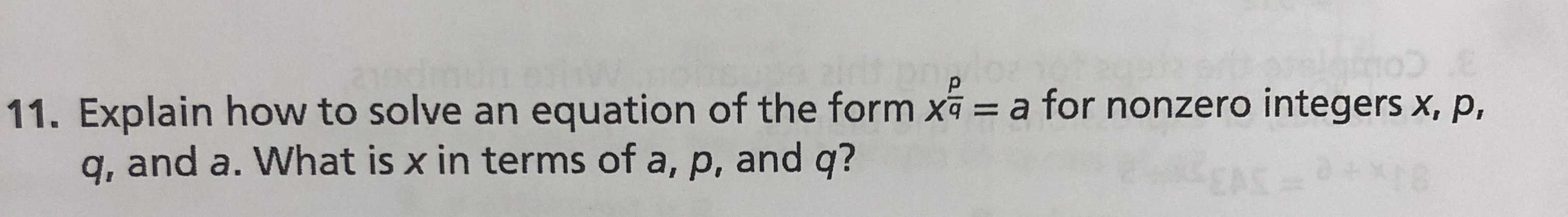### ¿Todavía tienes preguntas de matemáticas?

Pregunte a nuestros tutores expertos
Algebra
Pregunta11. Explain how to solve an equation of the form $$x ^ { \frac { p } { q } } = a$$ for nonzero integers $$x , p$$ ,

$$q ,$$ and $$a .$$ What is $$x$$ in terms of $$a , p ,$$ and $$q$$ ?

$$x= e^{\frac{q}{p}\ln{a}}$$